# Decision Tree in R : Step by Step Guide

This article explains the theoretical and practical application of decision tree with R. It covers terminologies and important concepts related to decision tree. In this tutorial, we run decision tree on credit data which gives you background of the financial project and how predictive modeling is used in banking and finance domain.

Decision Tree : Meaning

A decision tree is a graphical representation of possible solutions to a decision based on certain conditions. It is called a decision tree because it starts with a single variable, which then branches off into a number of solutions, just like a tree.

A decision tree has three main components :
1. Root Node : The top most node is called Root Node. It implies the best predictor (independent variable).
2. Decision / Internal Node : The nodes in which predictors (independent variables) are tested and each branch represents an outcome of the test
3. Leaf / Terminal Node : It holds a class label (category) - Yes or No (Final Classification Outcome).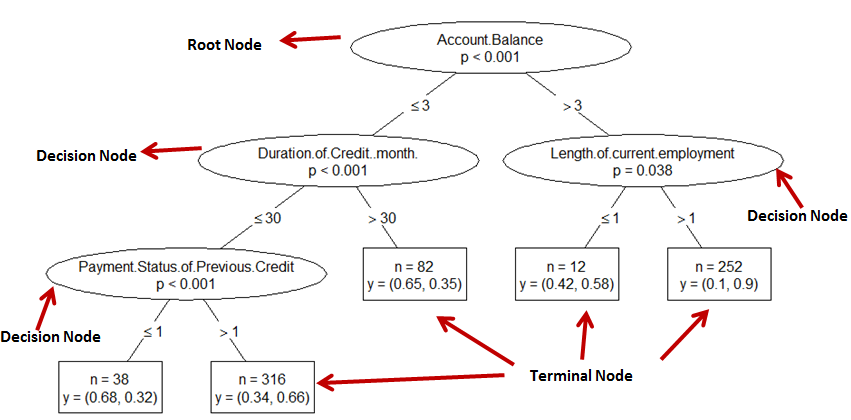Decision Tree Explained

1. Decision tree is easy to interpret.
2. Decision Tree works even if there is nonlinear relationships between variables. It does not require linearity assumption.
3. Decision Tree is not sensitive to outliers.

1. Decision tree model generally overfits. It means it does not perform well on validation sample.
2. It assumes all independent variables interact each other, It is generally not the case every time.

Terminologies related to decision tree

1. Pruning : Correct Overfitting

It is a technique to correct overfitting problem. It reduces the size of decision trees by removing sections of the tree that provide little power to classify instances. It is used to remove anomalies in the training data due to noise or outliers. The pruned trees are less complex trees.

Pre-Pruning Method : Significance Testing

It refers to the process in which we stop growing the tree when there is no statistically significant association between any attribute and the class at a particular node. The chi-squared test is used to check statistically significant association.

Post Pruning Method : Cost Complexity

Post pruning refers to the following process -
1. Build full tree
2. Prune it

The cost complexity is one of the most popular post-pruning method. It is measured by the following two parameters −
1. Number of leaves in the tree (i.e. size of the tree)
2. Error rate of the tree (i.e. misclassification rate or Sum of Squared Error)

The‘CP’ stands for Complexity Parameter of the tree. We want the cp value of the smallest tree that has smallest cross validation error. In regression, this means that the overall R-squared must increase by cp at each step.

In other words, it refers to trade-off between the size of a tree and the error rate to help prevent overfitting. Thus large trees with a low error rate are penalized in favor of smaller trees.
```        CP nsplit rel error  xerror     xstd
1 0.046948      0   1.00000 1.00000 0.057151
2 0.023474      4   0.75587 0.81221 0.053580
3 0.015649      5   0.73239 0.83099 0.053989
4 0.011737     10   0.64789 0.87324 0.054867
5 0.010955     12   0.62441 0.89671 0.055328
6 0.010000     17   0.56808 0.89671 0.055328
```

In this case, we pick the tree having CP = 0.023474 as it has least cross validation error (xerror). The rel error of each iteration of the tree is the fraction of misclassified cases in the iteration relative to the fraction of misclassified cases in the root node.
Cost Complexity (cp) is the tuning parameter in CART.

2. Splitting

It is a process of dividing a node into two or more sub-nodes.

3. Branch

A sub section of entire tree is called branch.

4. Parent Node

A node which splits into sub-nodes.

5. Child Node

It is the sub-node of a parent node.

6. Surrogate Split

When you have missing data, decision tree return predictions when they include surrogate splits. If parameter value of  surrogate is set 2, it means if the primary splitter is missing, we use the number one surrogate. If the number one surrogate is missing, then we use the number two surrogate.

Classification and Regression Tree (CART)

Classification Tree

The outcome (dependent) variable is a categorical variable (binary) and predictor (independent) variables can be continuous or categorical variables (binary).

How Decision Tree works:
1. Pick the variable that gives the best split (based on lowest Gini Index)
2. Partition the data based on the value of this variable
3. Repeat step 1 and step 2. Splitting stops when CART detects no further gain can be made, or some pre-set stopping rules are met. (Alternatively, the data are split as much as possible and then the tree is later pruned.

Algorithms of Classification Tree

1. Split Method : Gini Index

Gini Index measures impurity in node. It varies between 0 and (1-1/n) where n is the number of categories in a dependent variable.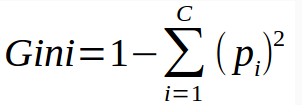Gini Index

In this equation, p refers to probability of class. In layman's language, it can be read as -
1 – ( P(class1)^2 + P(class2)^2 + … + P(classN)^2)
Gini Index favors larger partitions.

Important Points :
1. Zero.gini index implies perfect classification.
2. (1 - (1/ No. of classes) implies worst classification
3. We want a variable split having a low Gini Index.
4. For binary dependent variable, max gini index value can be 0.5. See the calculation below.
= 1 - (1/2)2 - (1/2)2
= 1 - 2*(1/2)2
= 1- 2*(1/4)
= 1-0.5
= 0.5

2. Entropy / Information Gain

Another splitting criteria method for classification tree is entropy. The formula of this technique is shown below -Entropy
It can be read as -
P(class1)*log(P(class1),2) + P(class2)*log(P(class2),2) + … + P(classN)*log(P(classN),2)
It favors partitions that have small counts but many distinct values.
Smaller value of Entropy signifies a good classification.

Information Gain can be calculated by using the following formula -
= Entropy(parent) - Weighted Sum of Entropy(Children)

Which is better - Entropy or Gini
Both splitting criterias are approximately similar and produces similar result in 95% of the cases. Gini is comparatively faster than Entropy as it does not require calculation of log.

Regression Tree
The outcome (dependent) variable is a continuous variable and predictor (independent) variables can be continuous or categorical variables (binary).

Split Method:  Least-Squared Deviation or Least Absolute Deviation

The impurity of a node is measured by the Least-Squared Deviation (LSD), which is simply the within variance for the node.

How to calculate best split manually

Suppose you have two independent variables that are continuous (numeric) in nature and target variable is binary which has only two values - 0/1. Sample data is shown below -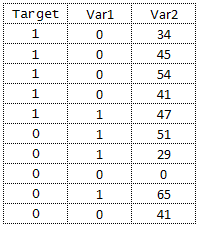Sample Data

Gini Index : Var1

Var1 = 1

1. Var1 has 4 cases out of 10 where it is equal to 1.
2. For Var1 = 1 & Target = 1, 1/4 cases have target=1.
3. For Var1 = 1 & Target = 0,  3/4 cases have target=0.
Gini Index = 1-((1/4)^2 + (3/4)^2) = 0.375

Var1 = 0

1. Var1 has 6 cases out of 10 where it is equal to 0.
2. For Var1 = 0 and Target = 1, 4/6 cases have target = 1.
3. For Var1 = 0 & Target = 0,  2/6 cases have target = 0.

Gini Index = 1-((4/6)^2 + (2/6)^2) = 0.4444

By adding weight and sum each of the gini indices:
Gini Index (Target, Var1) =  4/10 * 0.375 + 6/10 * 0.444 = 0.41667
Gini Index : Var2

For numeric variables, we first need to find the actual value as a threshold which gives the best split. There are k−1 possible splits on continuous variable , all of which should be used to determine an optimal split. Let's choose randomly 32 as a threshold.

Var2 >= 32

1. Var2 has 8 cases (8/10) where it is greater than or equal to 32.
2. For Var2 >= 32 and target = 1,  5/8 cases have target = 1.
3. For Var2 >= 32 & target = 0: 3 / 8 cases have target = 0.

Gini Index = 1-((5/8)^2 + (3/8)^2) = 0.46875

Var2 > 32

1. Var2 has 2 cases out of 10 where it is less than 32
2. For Var2 < 32 and target = 1, 0 cases have target = 1
3. For Var2 < 32 and target = 0,  2/2 cases have target = 0.

Gini Index = 1-((0/2)^2 + (2/2)^2) = 0
Gini Index(Target, Var2) = 8/10 * 0.46875 + 2/10 * 0 = 0.375
Since Var2 has lower Gini Index value, it should be chosen as a variable that gives best split. The next step would be to take the results from the split and further partition.  Let’s take the 8 / 10 cases and calculate Gini Index on the following 8 cases.

Target Var1 Var2
1 0 34
1 0 45
1 0 54
1 0 41
1 1 47
0 1 51
0 1 65
0 0 41

Try similar steps as what we performed earlier.

How to get predicted probability

Let' say an observation falls into lead node1 in which there are 6 class As and 4 class Bs, then probability P(ClassA | observation) = 6 / 10 = 0.6. Similarly, P(ClassB | observation) = 0.4.

Analysis of German Credit Data

The German Credit Data contains data on 20 variables and the classification whether an applicant is considered a Good or a Bad credit risk for 1000 loan applicants.
The objective of the model is whether to approve a loan to a prospective applicant based on his/her profiles.
1. Make sure all the categorical variables are converted into factors.
2. The function rpart will run a regression tree if the response variable is numeric, and a classification tree if it is a factor.
3. rpart parameter - Method - "class" for a classification tree ; "anova" for a regression tree
4. minsplit : minimum number of observations in a node before splitting. Default value - 20
5. minbucket : minimum number of observations in terminal node (leaf). Default value - 7 (i.e. minsplit/3)
6. xval : Number of cross validations
7. Prediction (Scoring) : If type = "prob": This is for a classification tree. It generates probabilities - Prob(Y=0) and Prob(Y=1).
8. Prediction (Classification) : If type = "class": This is for a classification tree. It returns 0/1.
R : Decision Tree

# Check attributes of data
str(mydata)
```'data.frame': 1000 obs. of  21 variables:
\$ Creditability                    : Factor w/ 2 levels "0","1": 2 2 2 2
\$ Account.Balance                  : int  1 1 2 1 1 1 1 1 4 2 ...
\$ Duration.of.Credit..month.       : int  18 9 12 12 12 10 8 6 18 24 ...
\$ Payment.Status.of.Previous.Credit: int  4 4 2 4 4 4 4 4 4 2 ...
\$ Purpose                          : int  2 0 9 0 0 0 0 0 3 3 ...
\$ Credit.Amount                    : int  1049 2799 841 2122 2171 2241
\$ Value.Savings.Stocks             : int  1 1 2 1 1 1 1 1 1 3 ...
\$ Length.of.current.employment     : int  2 3 4 3 3 2 4 2 1 1 ...
\$ Instalment.per.cent              : int  4 2 2 3 4 1 1 2 4 1 ...
\$ Sex...Marital.Status             : int  2 3 2 3 3 3 3 3 2 2 ...
\$ Guarantors                       : int  1 1 1 1 1 1 1 1 1 1 ...
\$ Duration.in.Current.address      : int  4 2 4 2 4 3 4 4 4 4 ...
\$ Most.valuable.available.asset    : int  2 1 1 1 2 1 1 1 3 4 ...
\$ Age..years.                      : int  21 36 23 39 38 48 39 40 65 23 ...
\$ Concurrent.Credits               : int  3 3 3 3 1 3 3 3 3 3 ...
\$ Type.of.apartment                : int  1 1 1 1 2 1 2 2 2 1 ...
\$ No.of.Credits.at.this.Bank       : int  1 2 1 2 2 2 2 1 2 1 ...
\$ Occupation                       : int  3 3 2 2 2 2 2 2 1 1 ...
\$ No.of.dependents                 : int  1 2 1 2 1 2 1 2 1 1 ...
\$ Telephone                        : int  1 1 1 1 1 1 1 1 1 1 ...
\$ Foreign.Worker                   : int  1 1 1 2 2 2 2 2 1 1 ...
```
# Check number of rows and columns
dim(mydata)

# Make dependent variable as a factor (categorical)
mydata\$Creditability = as.factor(mydata\$Creditability)

# Split data into training (70%) and validation (30%)
dt = sort(sample(nrow(mydata), nrow(mydata)*.7))
train<-mydata[dt,]
val<-mydata[-dt,] # Check number of rows in training data set
nrow(train)

# To view dataset
edit(train)

# Decision Tree Model
library(rpart)
mtree <- rpart(Creditability~., data = train, method="class", control = rpart.control(minsplit = 20, minbucket = 7, maxdepth = 10, usesurrogate = 2, xval =10 ))

mtree

#Plot tree
plot(mtree)
text(mtree)

#Beautify tree
library(rattle)
library(rpart.plot)
library(RColorBrewer)

#view1
prp(mtree, faclen = 0, cex = 0.8, extra = 1)

#view2 - total count at each node
tot_count <- function(x, labs, digits, varlen)
{paste(labs, "\n\nn =", x\$frame\$n)}

prp(mtree, faclen = 0, cex = 0.8, node.fun=tot_count)

#view3- fancy Plot
rattle()
fancyRpartPlot(mtree)

############################
########Pruning#############
############################

printcp(mtree)
bestcp <- mtree\$cptable[which.min(mtree\$cptable[,"xerror"]),"CP"]

# Prune the tree using the best cp.
pruned <- prune(mtree, cp = bestcp)

# Plot pruned tree
prp(pruned, faclen = 0, cex = 0.8, extra = 1)

# confusion matrix (training data)
conf.matrix <- table(train\$Creditability, predict(pruned,type="class"))
rownames(conf.matrix) <- paste("Actual", rownames(conf.matrix), sep = ":")
colnames(conf.matrix) <- paste("Pred", colnames(conf.matrix), sep = ":")
print(conf.matrix)

#Scoring
library(ROCR)
val1 = predict(pruned, val, type = "prob")
#Storing Model Performance Scores
pred_val <-prediction(val1[,2],val\$Creditability)

# Calculating Area under Curve
perf_val <- performance(pred_val,"auc")
perf_val

# Plotting Lift curve
plot(performance(pred_val, measure="lift", x.measure="rpp"), colorize=TRUE)

# Calculating True Positive and False Positive Rate
perf_val <- performance(pred_val, "tpr", "fpr")

# Plot the ROC curve
plot(perf_val, col = "green", lwd = 1.5)

#Calculating KS statistics
ks1.tree <- max(attr(perf_val, "y.values")[] - (attr(perf_val, "x.values")[]))
ks1.tree
```# Advanced Plot
prp(pruned, main="Beautiful Tree",
extra=106,
nn=TRUE,
fallen.leaves=TRUE,
branch=.5,
faclen=0,
trace=1,
branch.lty=3,
split.cex=1.2,
split.prefix="is ",
split.suffix="?",
split.box.col="lightgray",
split.border.col="darkgray",
split.round=.5)
```Beautiful Decision Tree
Related Posts
ShareDeepanshu founded ListenData with a simple objective - Make analytics easy to understand and follow. He has over 10 years of experience in data science. During his tenure, he worked with global clients in various domains like Banking, Insurance, Private Equity, Telecom and HR.

20 Responses to "Decision Tree in R : Step by Step Guide"
1.Nice Article! Thanks for making decision tree so simpler :-)

2.Remarkable and well defined

3.Nice Article. Could you please let me know how to calculate root mean error.

1.Sorry, I meant root node error.

4.Please provide decision tree in sas if you can, thanks

1.Decision tree algorithm is not available in SAS STAT. It is available in SAS Enterprise Miner. I don't have access to SAS Enterprise Miner. I can share some tutorial about how to build a decision tree in SAS Enterprise Miner if you want. Thanks!

5.amazing....

6.mtree <- rpart(Creditability~., data = train, method="class", control = rpart.control((minsplit = 20, minbucket = 7, maxdepth = 10, usesurrogate = 2, xval =10 ))
Error: unexpected ',' in "mtree <- rpart(Creditability~., data = train, method="class", control = rpart.control((minsplit = 20,"

I am getting this error can you please tell me the way, so that i don't get this error

1.There should be a single bracket in 'rpart.control(('. Use rpart.control( instead of rpart.control((. Let me know if it works. I am logged in via mobile. Will update the code in the article tomorrow.

7.Tree Lopping and Root Barriers could be considered cruel, an new way of practicing old behaviours but there is a place for it. The problem with having trees in your garden or in the street is that their roots eventually tear up the road and pavement or get into the foundations of your house. Look at this site

8.I have thought which I came across in beginning of tutorial with the mentioning of the "root" node. Isn't that the dependent variable from which mother and child node comes?Just asking to clear my doubt, because I see, that has been mentioned as the most important predictor.

1.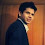The root node is the independent variable (predictor). In this example, the dependent variable is binary in nature - whether to approve a loan to a prospective applicant.

9.?Nice Article. Are you on github?

10.I am not able to understand below listed code nor you have provided complete explanation to the code/graphs

#Scoring
library(ROCR)
val1 = predict(pruned, val, type = "prob")
#Storing Model Performance Scores
pred_val <-prediction(val1[,2],val\$Creditability)

# Calculating Area under Curve
perf_val <- performance(pred_val,"auc")
perf_val

# Plotting Lift curve
plot(performance(pred_val, measure="lift", x.measure="rpp"), colorize=TRUE)

# Calculating True Positive and False Positive Rate
perf_val <- performance(pred_val, "tpr", "fpr")

# Plot the ROC curve
plot(perf_val, col = "green", lwd = 1.5)

Appreciate if you could please provide me an explanation.

1.pred is used in predicting the class codes(0,1)
the auc means the area under the roc curve
plot the performance means will plot the roc curve tpr against the fpr

11.Great Article ,provided clear cut concept.Thank you.

12.It will be better if you explain data too. Without understanding input data, this becomes mathematical exercise using R.

13.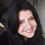I have a question. It is clear if we have n variables, the variable with the help of which we will get minimum GINI split, the code will use that variable to split the tree. But what if we get only a ROOT node as the output- as in which value is considered as the reference point to say that beyond the root node, there is no point of splitting as impurity will increase. We are comparing GINI split of all dependent variable with which value to arrive at this conclusion.

14.1.Please confirm why we should convert categorical var to factor in decision tree
2.how to do pre pruning

15.I loved the article (a big thank you),But I am not being able install these libraries.
when I trying to install these libraries it giving me these errors
"install.packages(rattle)